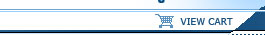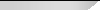NOTICE: TITANCE.COM WILL BE DOWN FOR SCHEDULED MAINTENANCE BEGINNING AT 6:00PM ET ON WEDNESDAY, JULY 24TH.Online CoursesContractors/TradesArchitectsEngineersHome Study Course BooksContractors/TradesArchitectsEngineersLive WebinarsArchitectsEngineersLive SeminarsContractors/TradesArchitectsEngineers
 If you have any questions please call a Titan Representative 1-800-960-8858

Pipe Flow Calculations Part I - Friction Factor Calculations with Excel (3 credit hours)
Several kinds of pipe flow calculations can be made with the Darcy-Weisbach equation and the Moody friction factor.  These calculations can be conveniently carried out with an Excel spreadsheet.  Many of the calculations require an iterative solution, so they are especially suitable for an Excel spreadsheet solution.  This 3 hour course includes discussion of the Darcy-Weisbach equation and the parameters in the equation along with the U.S. and S.I. units to be used.  Example calculations and sample Excel spreadsheets for making the calculations are also presented and discussed.

This 3 hour course is intended primarily for civil engineers, mechanical engineers, chemical engineers, and environmental engineers.  After completing this course you will be able to make calculations with the Darcy Weisbach equation and the Moody friction factor equations to calculate several different unknown parameters when sufficient input data is provided.  You will also be prepared to use Excel spreadsheets to efficiently make the calculations.

As prepared for Titan Coursework this series has been separated into separate courses and may be taken out of sequence, each course independent from another.
 Harlan Bengtson
• Be able to calculate the Reynolds number for pipe flow with specified flow conditions
• Be able to determine whether a specified pipe flow is laminar or turbulent flow for specified flow conditions
• Be able to calculate the entrance length for pipe flow with specified flow conditions
• Be able to obtain a value for the friction factor using the Moody diagram for given Re and e/D.
• Be able to calculate a value for the friction factor for specified Re and e/D, using the appropriate equation for f.
• Be able to determine a value of the Moody friction factor from the Moody diagram, for given Re and e/D.
• Be able to calculate a value of the Moody friction factor for given Re and e/D, using the Moody friction factor equations.
• Be able to use the Darcy Weisbach equation and the Moody friction factor equations to calculate the frictional head loss and frictional pressure drop for a given flow rate of a specified fluid through a pipe with known diameter, length and roughness.
• Be able to use the Darcy Weisbach equation and the Moody friction factor equations to calculate the required diameter for a given flow rate of a specified fluid through a pipe with known length and roughness, with specified allowable head loss.
• Be able to use the Darcy Weisbach equation and the Moody friction factor equations to calculate the fluid flow rate through a pipe with known diameter, length and roughness, with specified frictional head loss.
As prepared for Titan Coursework this series has been separated into separate courses and may be taken out of sequence, each course independent from another.Titan Continuing Education, Inc. | 1519 Dale Mabry Hwy, Ste 201 Lutz, FL 33548 | Toll Free: 800.960.8858 | Email: info@TitanCE.com.